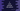# C program to print pyramid using star or any other character### C program to print a pyramid using star or any other character :

In this tutorial, we will learn how to print a pyramid using star ’*’ or any other character using ‘C programming language’. The user will input the height of the pyramid and we will print the pyramid equal to that height. It will look like as below :

``````    *
***
*****
*******
*********
``````

The height of this pyramid is 5. So, how is it working?

### How the program works :

We are printing blank space and * to print this full pyramid. The height of the pyramid is 5. If we change the program to print # instead of space, it will look like :

``````####*
###***
##*****
#*******
*********
``````

Think # as space and height is 5 . If we analyze the above pyramid, we can find out that :

1. First line: four # and one *. That means we will print four spaces and one * for the first line if height is 5.
2. Second line: three # and three *. That means we will print three spaces and three * for the second line if height is 4
3. From the above two lines, it is clear that we will print space for n times. n is the row number for the pyramid. For the first row, we are printing # for one time, for the second row, we are printing # two times, etc.
4. After printing space, we will print * for 2*n - 1 time. Where n is the row number. For the first row, we are printing 2 * 1 - 1 = 1 time. For second-line, we will print * for 2 * 2 - 1 = 3 times, etc.
5. To print the full pyramid, we will use one for loop. The loop will run from 1 to the height of the pyramid. Using the above two methods, we will print the full pyramid.

### C program :

``````#include <stdio.h>

int main()
{
int i, j, height;

printf("Enter height of the pyramid : \n");
scanf("%d", &height);

for (i = 1; i <= height; i++)
{

for (j = i; j < height; j++)
{
printf(" ");
}

for (j = 1; j <= (2 * i - 1); j++)
{
printf("*");
}

printf("\n");
}

return 0;
}``````

### Example Output :

``````Enter height of the pyramid :
5
*
***
*****
*******
*********

Enter height of the pyramid :
10
*
***
*****
*******
*********
***********
*************
***************
*****************
*******************``````